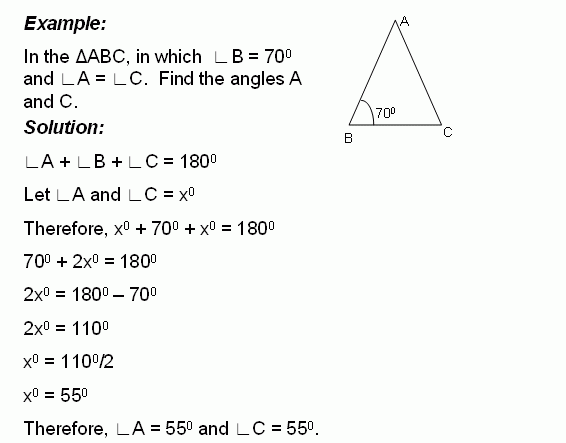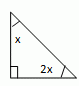Name: ___________________Date:___________________

 Email us to get an instant 20% discount on highly effective K-12 Math & English kwizNET Programs!

### Middle/High School Algebra, Geometry, and Statistics (AGS)6.14 Find the Angles of TriangleDirections: Solve the following problems. Also write at least 10 examples of your own.Name: ___________________Date:___________________

### Middle/High School Algebra, Geometry, and Statistics (AGS)6.14 Find the Angles of Triangle

 Q 1: Find the angles of the triangle and classify based on the angles.32, 96, right angle triangle26, 64, right angle triangle30, 60, right angle triangle Q 2: Find the angles of the triangle.45, 45, 90 triangle60, 60, 60 triangle30, 60, 90 triangle Question 3: This question is available to subscribers only! Question 4: This question is available to subscribers only!